Courses

# Test: Thermodynamics

## 25 Questions MCQ Test Chemistry for JEE | Test: Thermodynamics

Description
This mock test of Test: Thermodynamics for JEE helps you for every JEE entrance exam. This contains 25 Multiple Choice Questions for JEE Test: Thermodynamics (mcq) to study with solutions a complete question bank. The solved questions answers in this Test: Thermodynamics quiz give you a good mix of easy questions and tough questions. JEE students definitely take this Test: Thermodynamics exercise for a better result in the exam. You can find other Test: Thermodynamics extra questions, long questions & short questions for JEE on EduRev as well by searching above.
QUESTION: 1

### If Vf is the final volume and Vi is the initial volume and pex the external pressure the work done can be calculated by

Solution:

Work done =- ViVf pext dVwhere Vi is initial and Vf is final volume. Pex is the external pressure applied on system while change in volume

QUESTION: 2

### Spontaneity in the context of chemical thermodynamics means

Solution:

Spontaneity means a reaction occurring on its own without any help of external agency.

QUESTION: 3

### Enthalpy of combustion of carbon to CO2is –393.5 kJ mol−1. Calculate the heat released upon formation of 35.2 g of CO2from carbon and dioxygen gas.

Solution:

when 1 mole of CO2 is produced energy released is –393.5 kJ mol−1 Moles of CO2 given =35.2/44 =0.8 moles So energy released = 0.8 x393.5 KJ/mol = 315 KJ/mol

QUESTION: 4

The volume of gas is reduced to half from its original volume. The specific heat will

Solution:

Specific heat will remain constant.

QUESTION: 5

A spherical constant temperature heat source of radius r1 is at the center of a uniform solid sphere of radius r2. The rate at which heat is transferred through the surface of the sphere is proportional to

Solution:

The rate H at which heat is transferred through the slab is,

(a) directly proportional to the area (A) available.

(b) inversely proportional to the thickness of the slab Δx.

(c) directly proportional to the temperature difference ΔT.

So, H = kA ΔT/ Δx

Where k is the proportionality constant and is called thermal conductivity of the material.

From above we know that the rate H at which heat is transferred through the slab is directly proportional to the area (A) available.

Area A of the solid sphere is defined as,

A = 4πr2

Here r is the radius of the sphere.

So, the area A1 of a uniform small solid sphere having radius r1 will be,

A1 = 4πr12

And, the area A2 of a uniform large solid sphere having radius r2 will be,

A2 = 4πr22

Thus the area A from which heat is transferred through the surface of the sphere will be the difference of area of uniform large solid sphere A2 and small solid sphere A1.

So, A = A2 - A= 4πr22 - 4πr1= 4π (r22 - r12)

Since the rate H at which heat is transferred through the slab is directly proportional to the area (A) available, therefore the rate at which heat is transferred through the surface of the sphere is proportional to r22 - r12.

QUESTION: 6

A cylinder confines 2.00 L gas under a pressure of 1.00 atm. The external pressure is also 1.00 atm. The gas is heated slowly, with the piston sliding freely outward to maintain the pressure of the gas close to 1.00 atm. Suppose the heating continues until a final volume of 3.50 L is reached. Calculate the work done on the gas.

Solution: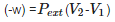QUESTION: 7

Enthalpy is defined as

Solution:

H =U+pV. H is enthalpy of reaction.

QUESTION: 8

Entropy is a state function and measures

Solution:

Entropy is a state function and it is measure of randomness of a system.

QUESTION: 9

For a process from state 1 to state 2, heat transfer in a reversible process is given by

Solution:

Qreversible = T∆S
Hence, Q = To(S2-S1)

QUESTION: 10

During complete combustion of one mole of butane, 2658 kJ of heat is released. The thermochemical reaction for above change is ΔfUof formation of CH4 (g) at certain temperature is –393 kJ mol−1. The value of ΔfH0is

Solution:

C4H10(g) + 13/2O2  →  4CO2(g) + 5H2O(l)
∆Ng = (ng)product - (ng)reactant
= 4-(13/2+1) = -7/2
or ∆Ng is negative.
∆H = ∆U + ∆NgRT
SInce ∆ng is negative therefore ∆H is less than ∆U.

QUESTION: 11

Suppose a 10.00-kg mass drops through a height difference of 3.00 m, and the resulting work is used to turn a paddle in 200.0 g water, initially at 15.00C. The final water temperature is found to be 15.35C. Assuming that the work done is used entirely to increase the water temperature, calculate the conversion factor between joules and calories.

Solution:

1 calorie≈4.2J

QUESTION: 12

The molar heat capacity of a substance is the

Solution:

The molar heat capacity of a substance is the quantity of heat needed to raise the temperature of one mole by one degree Celsius.

QUESTION: 13

If change in Gibbs energy ΔG is negative (< 0)

Solution:

ΔG < 0 means reaction is spontaneous. And ΔG >0 means reaction is non spontanenous.

QUESTION: 14

Calculate the standard enthalpy of formation of CH3OH(l) from the following data:

CH3​OH(I)+3/2​O2​(g)→CO2​(g)+2H2​O(l);  △r​Hθ=−726kJmol−1

C(graphite)​+O2​(g)→CO2​(g);    △c​Hθ=−393kJmol−1

H2​(g)+1/2​O2​(g)→H2​O(l);    △f​Hθ=−286kJmol−1

Solution: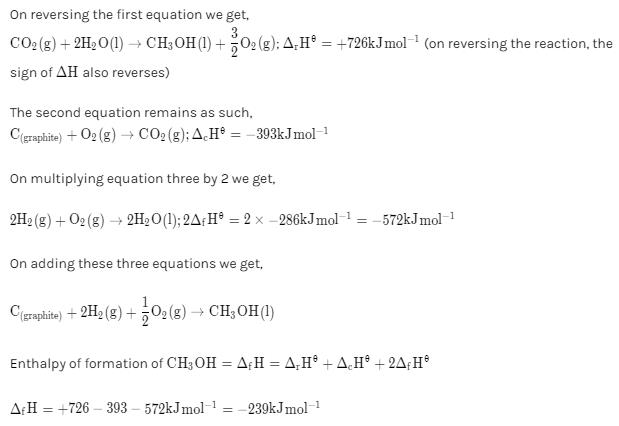QUESTION: 15

In an adiabatic process, no transfer of heat takes place between system and surroundings. Choose the correct option for free expansion of an ideal gas under adiabatic condition from the following.

Solution:

for free expansion Pext =0 so w=0 and for adiabatic process q=0 therefore ΔU =0 making ΔT=0

QUESTION: 16

Standard Molar Enthalpy of Formation is

Solution:

The standard enthalpy change for the formation of one mole of a compound from its elements in their most stable states of aggregation is enthalpy of formation.

QUESTION: 17

The relationship between Cp and CV for an ideal gas is

Solution:

For ideal gas Cp - CV = nR. For1 mole of gas n=1.

QUESTION: 18

A thermodynamic state function is a physical quantity

Solution:

State function is one which is only dependent on initial and final state of the system and is independent of the path by which that change has occurred.

QUESTION: 19

Solution:

Thermodynamics is not concerned about how and at what rate these energy transformations are carried out, but is based on initial and final states of a system undergoing the change. Laws of thermodynamics apply only when a system is in equilibrium or moves from one equilibrium state to another equilibrium state.

QUESTION: 20

The pressure-volume work for an ideal gas can be calculated by using the expression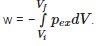The work can also be calculated from the pV– plot by using the area under the curve within the specified limits. When an ideal gas is compressed (a) reversibly or (b) irreversibly from volume Vi to Vf . choose the correct option.

Solution:

w (reversible) < w (irreversible) (for compression process)

• Work done is the area under the P−V curve. It can be seen in the curve above that the area under the P−V curve for irreversible compression of the gas is more than the area under the curve for reversible compression.
• Thus, work done for irreversible compression is more than that for reversible compression.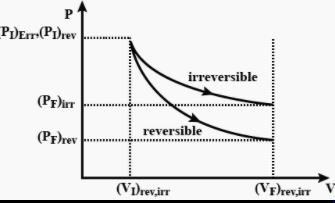QUESTION: 21

Suppose that 1.00 kJ of heat is transferred to 2.00 mol argon (at 298 K, 1 atm). What will the final temperature Tf be if the heat is transferred at constant volume?

Solution:

since volume is constant so w=0 so q=ΔE=nCvΔT Cv=(3/2)R

Because  argon is monoatomic, approximately ideal gas,

cv = 3/2 R = 12.47 J K-1 mol-1

At constant volume,

q= n cv Δ T

1000J = (2.00 mol)(12.47 JK-1mol-1)ΔT

ΔT = 40.1 k Tf = 298 + 40.1 =338 K

QUESTION: 22

An exothermic process

Solution:

Exothermic process results in decrease in enthalpy of system because for exothermic reaction ΔH=-ve.

QUESTION: 23

For the process to occur under adiabatic conditions, the correct condition is:

Solution:

QUESTION: 24

For an isolated system, ΔU = 0, what will be ΔS?

Solution:

An isolated system is that system which can neither do work nor can take/give heat from or to the surrounding.
In this type of system,
dU=dQ=0,
since
dS=dQ/dt
dS=0

QUESTION: 25

The entropy change can be calculated by using the expression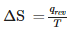When water freezes in a glass beaker, choose the correct statement amongst the following :

Solution:

For freezing of process since process is spontaneous therefore if ΔS (system) decreases but ΔS (surroundings) increases.